Next: Worked example 10.3: Leaning Up: Statics Previous: Worked example 10.1: Equilibrium

## Worked example 10.2: Rod supported by a cable

Question: A uniform rod of mass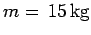and length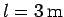is supported in a horizontal position by a pin and a cable, as shown in the figure below. Masses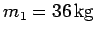and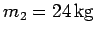are suspended from the rod at positions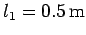and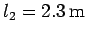. The angleis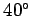. What is the tension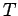in the cable?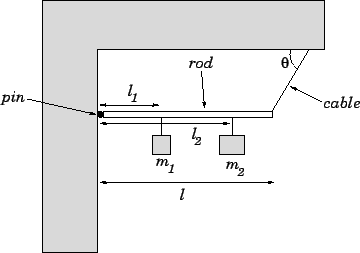Answer: Consider the torque acting on the rod about the pin. Note that the reaction at the pin makes no contribution to this torque (since the length of the associated lever arm is zero). The torque due to the weight of the rod is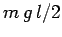(i.e., the weight times the length of the lever arm). Note that the weight of the rod acts at its centre of mass, which is located at the rod's mid-point. The torque due to the weight of the first mass is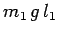. The torque due to the weight of the second mass is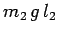. Finally, the torque due to the tension in the cable is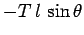(this torque is negative since it twists the rod in the opposite sense to the other three torques). Hence, setting the net torque to zero, we obtain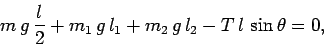or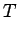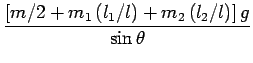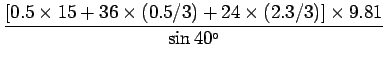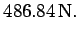Next: Worked example 10.3: Leaning Up: Statics Previous: Worked example 10.1: Equilibrium
Richard Fitzpatrick 2006-02-02